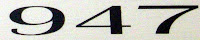## Monday, January 24, 2011

### 947

947 is a prime number. The difference between any two of its digits is also a prime.

947 is a Chen prime because 947 + 2 = 949 = 13 x 73, so 949 is a semiprime.

947 is the maximum number of regions into which 43 lines divide the plane.

947 is the sum of seven consecutive primes: 947 = 113 + 127 + 131 + 137 + 139 + 149 + 151.947 is the telephone area code for Oakland, Michigan. Note that the words "telephone code Oakland" have, respectively, 9, 4, and 7 digits.